Skip to content
Related Articles
Predictive Parser in Compiler Design
• Last Updated : 18 Mar, 2021

In this, we will cover the overview of Predictive Parser and mainly focus on the role of Predictive Parser. And will also cover the algorithm for the implementation of the Predictive parser algorithm and finally will discuss an example by implementing the algorithm for precedence parsing. Let’s discuss it one by one.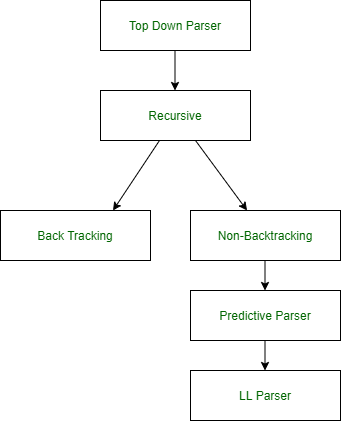Predictive Parser :
A predictive parser is a recursive descent parser with no backtracking or backup. It is a top-down parser that does not require backtracking. At each step, the choice of the rule to be expanded is made upon the next terminal symbol.
Consider

`A -> A1 | A2 | ... | An`

If the non-terminal is to be further expanded to ‘A’, the rule is selected based on the current input symbol ‘a’ only.

Predictive Parser Algorithm :

1. Make a transition diagram(DFA/NFA) for every rule of grammar.
2. Optimize the DFA by reducing the number of states, yielding the final transition diagram.
3. Simulate the string on the transition diagram to parse a string.
4. If the transition diagram reaches an accept state after the input is consumed, it is parsed.

Consider the following grammar –

```E->E+T|T
T->T*F|F
F->(E)|id```

After removing left recursion, left factoring

```E->TT'
T'->+TT'|ε
T->FT''
T''->*FT''|ε
F->(E)|id```

STEP 1:
Make a transition diagram(DFA/NFA) for every rule of grammar.

• E->TT’• T’->+TT’|ε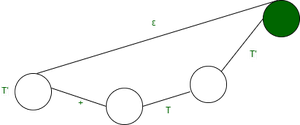• T->FT”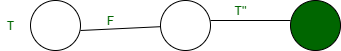• T”->*FT”|ε• F->(E)|idSTEP 2:
Optimize the DFA by decreases the number of states, yielding the final transition diagram.

• T’->+TT’|ε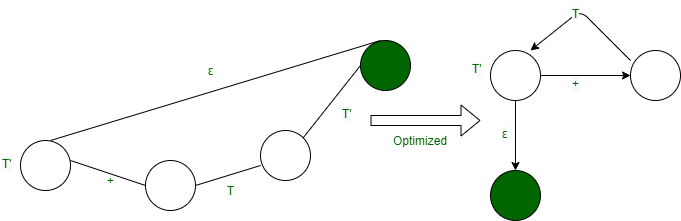It can be optimized ahead by combining it with DFA for E->TT’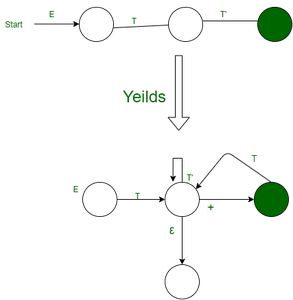Accordingly, we optimize the other structures to produce the following DFA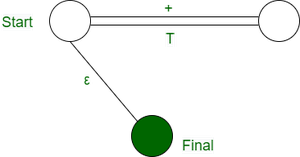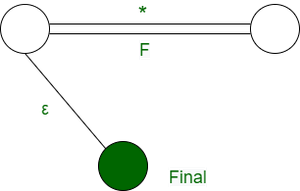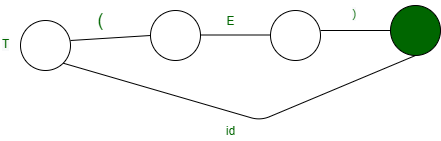STEP 3:
Simulation on the input string.
Steps involved in the simulation procedure are:

1. Start from the starting state.
2. If a terminal arrives consume it, move to the next state.
3. If a non-terminal arrive go to the state of the DFA of the non-terminal and return on reached up to the final state.
4. Return to actual DFA and Keep doing parsing.
5. If one completes reading the input string completely, you reach a final state, and the string is successfully parsed.

Attention reader! Don’t stop learning now. Learn all GATE CS concepts with Free Live Classes on our youtube channel.

My Personal Notes arrow_drop_up